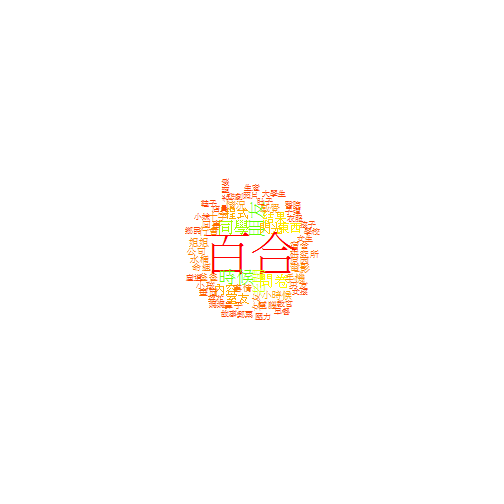# 用R進行中文 text Mining

Kaohsiung useR! Meetup Taiwan R User Group

### 安裝需要工具

``````install.packages("rJava")
install.packages("Rwordseg", repos="http://R-Forge.R-project.org")
install.packages("tm")
install.packages("tmcn", repos="http://R-Forge.R-project.org", type="source")
install.packages("wordcloud")
install.packages("XML")
install.packages("RCurl")
``````

Windows上安裝rJava的注意事項:

• 將jvm.dll加到環境變數PATH之中
• 注意java的版本(32-bit or 64-bit)必須要和R一致 (Windows binary)之後再安裝，以避免

### 抓取ptt笨版文章列表

``````library(XML)
library(RCurl)

data <- list()

for( i in 1058:1118){
tmp <- paste(i, '.html', sep='')
url <- paste('www.ptt.cc/bbs/StupidClown/index', tmp, sep='')
html <- htmlParse(getURL(url))
url.list <- xpathSApply(html, "//div[@class='title']/a[@href]", xmlAttrs)
data <- rbind(data, paste('www.ptt.cc', url.list, sep=''))
}
data <- unlist(data)

``````

``````getdoc <- function(line){
start <- regexpr('www', line)
end <- regexpr('html', line)

if(start != -1 & end != -1){
url <- substr(line, start, end+3)
html <- htmlParse(getURL(url), encoding='UTF-8')
doc <- xpathSApply(html, "//div[@id='main-content']", xmlValue)
name <- strsplit(url, '/')[]
write(doc, gsub('html', 'txt', name))
}
}

``````

``````sapply(data, getdoc)

``````

### 開始文字處理

``````library(tm)
library(tmcn)
``````
``````## # tmcn Version: 0.1-2
``````
``````library(Rwordseg)
``````
``````## Loading required package: rJava
## # Version: 0.2-1
``````

``````d.corpus <- Corpus(DirSource("doc"), list(language = NA))
``````

### 進行數據清理

1 清除標點符號, 數字

``````d.corpus <- tm_map(d.corpus, removePunctuation)
d.corpus <- tm_map(d.corpus, removeNumbers)
``````

2 清除大小寫英文與數字

``````d.corpus <- tm_map(d.corpus, function(word) {
gsub("[A-Za-z0-9]", "", word)
})
``````

### 進行中文斷詞

``````words <- readLines("http://wubi.sogou.com/dict/download_txt.php?id=9182")
insertWords(words)
``````

``````d.corpus <- tm_map(d.corpus[1:100], segmentCN, nature = TRUE)
d.corpus <- tm_map(d.corpus, function(sentence) {
noun <- lapply(sentence, function(w) {
w[names(w) == "n"]
})
unlist(noun)
})
d.corpus <- Corpus(VectorSource(d.corpus))
``````

``````myStopWords <- c(stopwordsCN(), "編輯", "時間", "標題", "發信", "實業", "作者")
d.corpus <- tm_map(d.corpus, removeWords, myStopWords)
``````

``````head(myStopWords, 20)
``````
``````##   "第二"     "一番"     "一直"     "一<U+4E2A>" "一些"     "<U+8BB8>多"
##   "种"       "有的是"   "也就是<U+8BF4>" "末"       "啊"       "阿"
##  "哎"       "哎呀"     "哎<U+54DF>" "唉"       "俺"       "俺<U+4EEC>"
##  "按"       "按照"
``````

### 建立 TermDocumentMatrix

``````tdm <- TermDocumentMatrix(d.corpus, control = list(wordLengths = c(2, Inf)))
``````

``````inspect(tdm[1:10, 1:2])
``````
``````## A term-document matrix (10 terms, 2 documents)
##
## Non-/sparse entries: 3/17
## Sparsity           : 85%
## Maximal term length: 3
## Weighting          : term frequency (tf)
##
##         Docs
## Terms    M.1384834727.A.0CB.txt M.1384838698.A.957.txt
##   一生                        0                      0
##   一家                        1                      0
##   一家子                      0                      0
##   一線                        0                      0
##   人士                        0                      0
##   人才                        0                      0
##   人中                        0                      0
##   人文                        0                      0
##   人民                        0                      1
##   人生                        0                      2
``````

### 畫出關鍵字詞雲

``````library(wordcloud)
``````
``````m1 <- as.matrix(tdm)
v <- sort(rowSums(m1), decreasing = TRUE)
d <- data.frame(word = names(v), freq = v)
wordcloud(d\$word, d\$freq, min.freq = 10, random.order = F, ordered.colors = F,
colors = rainbow(length(row.names(m1))))
``````### 尋找關鍵字之間的關聯

``````d.dtm <- DocumentTermMatrix(d.corpus, control = list(wordLengths = c(2, Inf)))
inspect(d.dtm[1:10, 1:2])
``````
``````## A document-term matrix (10 documents, 2 terms)
##
## Non-/sparse entries: 1/19
## Sparsity           : 95%
## Maximal term length: 2
## Weighting          : term frequency (tf)
##
##                         Terms
## Docs                     一生 一家
##   M.1384834727.A.0CB.txt    0    1
##   M.1384838698.A.957.txt    0    0
##   M.1384840050.A.414.txt    0    0
##   M.1384840304.A.EF5.txt    0    0
##   M.1384842495.A.5B8.txt    0    0
##   M.1384842609.A.A5B.txt    0    0
##   M.1384847473.A.6A5.txt    0    0
##   M.1384847771.A.729.txt    0    0
##   M.1384849471.A.B71.txt    0    0
``````

``````findFreqTerms(d.dtm, 30)
``````
``````##  "同學" "百合" "老闆" "朋友" "東西" "時候" "閃光" "問卷" "結果"
``````

``````findAssocs(d.dtm, "同學", 0.5)
``````
``````## 同學.原因
##      0.56
``````

### 參考資料

MLDM Monday - 如何用 R 作中文斷詞

using-the-rjava-package-on-win7-64-bit-with-r